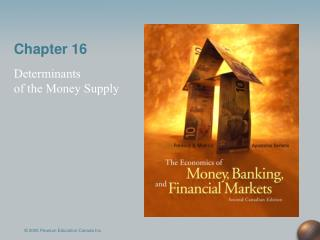DownloadDownload PresentationChapter 16

# Chapter 16

Download Presentation## Chapter 16

- - - - - - - - - - - - - - - - - - - - - - - - - - - E N D - - - - - - - - - - - - - - - - - - - - - - - - - - -
##### Presentation Transcript

1. Chapter 16 Determinants of the Money Supply © 2005 Pearson Education Canada Inc.

2. The Simple Deposit Multiplier from Chapter 15 Simple Deposit Multiplier 1 D = R r Deriving the formula R = DR = rD 1 D = R r 1 D = R r © 2005 Pearson Education Canada Inc.

3. Critique of the Simple Model The simple model of multiple deposit creation shows how the Bank of Canada can control D by setting R. That simple model however, ignores 1. the public’s decisions regarding how much C to hold, • the banks’ decisions regarding the amount of R they wish to hold, and • borrowers’ decisions on how much to borrow from banks. Recall also that the Bank of Canada can exert more precise control over MB ( = C + R) than over R. © 2005 Pearson Education Canada Inc.

4. The Money Supply Model Because the Bank of Canada can exert more precise control over MB than it can over R, in Chapter 16 we derive a multiplicative relation between M and MB, M = mMB, where m is the money multiplier. m relates the change in M to a given change in MB. © 2005 Pearson Education Canada Inc.

5. Money Multiplier M = mMB Deriving Money Multiplier R = DR DR = rD R = (rD) Adding C to both sides R + C = MB = C + (rD) 1. Tells us amount of MB needed support D and C 2. An extra \$1 of MB that arises from an extra \$1 of C does not support any additional D. That is, the C component of MB does not lead to a multiple deposit creation as the R component does. © 2005 Pearson Education Canada Inc.

6. (Continued) To put it differently, An  in MB that goes into C is not multiplied, whereas an  that goes into supporting deposits is multiplied. We have MB = C + (rD) To deal with currency drains, we also assume that C = c  D where c is the currency ratio. Hence, MB = (c  D) + (r D) = (c + r ) D © 2005 Pearson Education Canada Inc.

7. M = (cD) + D = (1 + c) D m < 1/r because no multiple expansion for currency and because as DER © 2005 Pearson Education Canada Inc.

8. An Example r= 5% C = \$40 billion D = \$160 billion M (= M1+) = C + D = \$200 billion The money multiplier tells us that given the behavior of the public as represented by c = 0.25 and that of banks as represented by r= 5%, a \$1  in MB will lead to a \$4.2  in M. If c = 0 (no currency drains), then m = 20 . © 2005 Pearson Education Canada Inc.

9. (Continued) Also notice that This is the deposit multiplier when c = 25% and r= 5%. It tells us that given the behavior of the public as represented by c = 25% and that of banks as represented by r= 5%, a \$1  in MB will lead to a \$3.3  in D. © 2005 Pearson Education Canada Inc.

10. The Full Model So far we have been assuming that the Bank has complete control over MB. This is not the case. The Bank lacks complete control over MB because it cannot unilaterally determine the amount of borrowing by banks from the Bank. Here we split MB into two components: • nonborrowed monetary base (MBn). This component of the MB is directly under the Bank’s control because it results from open market operations. • borrowed monetary base (or advancesA). This is the less tightly controlled component of the base, because it is influenced by banks’ decisions. © 2005 Pearson Education Canada Inc.

11. (Continued) Hence, MB = MBn + A To complete the money supply model, we rewrite it as M = mMB = m(MBn + A) where m is defined as before. Thus in addition to the effects on M of c and r, the expanded model stipulates that M is also affected by changes in MBn and A. In fact, because m > 0, there is a positive relation between M and each of MBn and A. © 2005 Pearson Education Canada Inc.

12. Overview of the Money Supply Process M = m(MBn + A) Open market purchases MBn and for given c, r, and A lead to an  in M. Open market sales MBn and for given c, r, and A lead to a  in M. Hence M is positively related to MBn. For given MBn , c, and r, an  in A will MB and lead to a multiple  in M. For given MBn , c, and r, an  in A will MB and lead to a multiple  in M. Hence M is positively related to A. © 2005 Pearson Education Canada Inc.